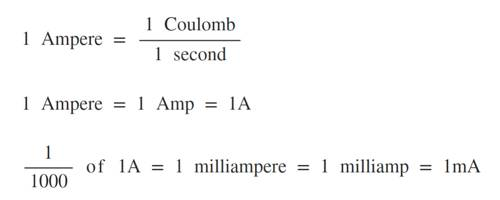# One Ampere

One ampere: Ampere or amp (symbol: A) is the unit of electrical current. The amount of current flowing in each of the two parallel wires of a negligible cross-section and of infinite length for which magnetic force of 2 x 10-7 N is produced per unit length of both the wires is called one ampere. One Ampere of current means one Coulomb of charge flowing through a conductor in one second. The ampere is named after Andre Marie Ampere, French physicist (1775-1836).An ampere is a unit of measure of the rate of electron flow or current in an electrical conductor. One ampere of current represents one coulomb of electrical charge (6.24 x 1018 charge carriers) moving past an exact point in one second. In practical terms, the ampere is a calculate of the amount of electric charge passing a point in an electric circuit per unit time with 6.241 × 1018 electrons, or one coulomb per second constituting one ampere.